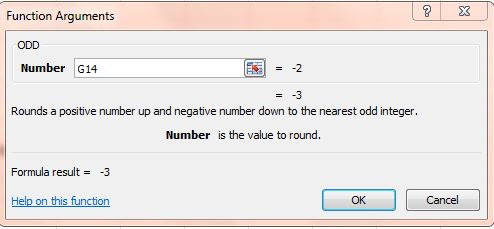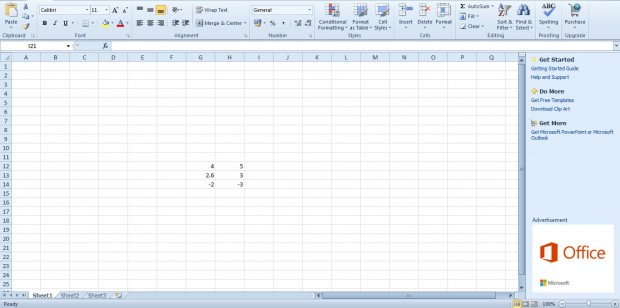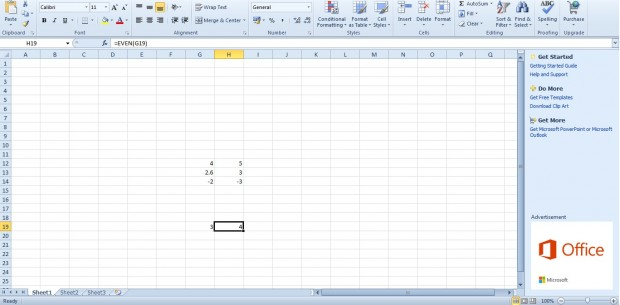# How to use ODD and EVEN functions in Excel spreadsheets [Tip]

Excel has numerous functions that you can add to spreadsheets. Among them are ODD and EVEN. Those are functions that round numbers to the closest odd or even values.

To add the ODD function to spreadsheets, click the fx button. Then select ODD from the Insert Function window. That will open the the function’s window below.This is a basic function that requires a cell reference. So select a cell, or range of cells, that include the values by pressing the button beside the Number field. Then press OK to close the window.

That will add the function to spreadsheet as shown below. Note that it rounds the number up to the nearest odd number. So four is rounded up to five and not three. However, if the number is below zero it is rounded down to the nearest odd value. If the cell includes a real number, a value with fractional component, it rounds to an integer.You can also add the EVEN function much the same. Click a cell to add the function to and press fx. Select EVEN from the Insert Function window. Then press the button beside Number and select the cells to round. The function rounds the cell values to even numbers as below.They are handy functions for rounding numbers to integers. For further details, head over to this YouTube page that includes an ODD/EVEN Excel function video.